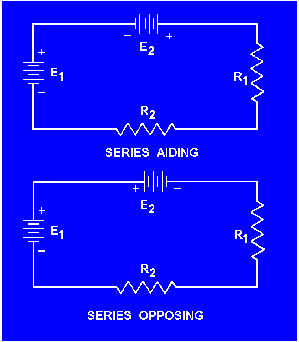voltages are added. Sources of emf that would tend to force current in opposite directions are said to be SERIES OPPOSING, and the effective source voltage is the difference between the opposing voltages."> Series Aiding and Opposing SourcesCustom SearchApplication of Kirchhoff's voltage lawMatter, Energy, and ElectricityCircuit terms and characteristicsSeries Aiding and Opposing Sources
In many practical applications a circuit may contain more than one source of emf. Sources of emf that cause current to flow in the same direction are considered to be SERIES AIDING and the voltages are added. Sources of emf that would tend to force current in opposite directions are said to be SERIES OPPOSING, and the effective source voltage is the difference between the opposing voltages. When two opposing sources are inserted into a circuit current flow would be in a direction determined by the larger source. Examples of series aiding and opposing sources are shown in figure 3-26.

Figure 3-26. - Aiding and opposing sources.A simple solution may be obtained for a multiple-source circuit through the use of Kirchhoff's voltage law. In applying this method, the same procedure is used for the multiple-source circuit as was used above for the single-source circuit. This is demonstrated by the following example.

Example: Using Kirchhoff's voltage equation, find the amount of current in the circuit shown in fig 3-27.

Figure 3-27.-Solving for circuit current using Kirchhoff's voltage equation.Solution: As before, a direction of current flow is assumed and polarity signs are placed on the drawing. The loop equation will be started at point A.

E2 + ER1 + E1 + E3 + ER2 = 0When using Kirchhoff's voltage law, how are voltage polarities assigned to the voltage drops across resistors?

Refer to figure 3-27, if R1was
changed to a 40-ohm resistor, what would be the value of circuit current (IT)?

Refer to figure 3-27. What is the effective source voltage of the circuit using the 40-ohm resistor?

Privacy Statement - Copyright Information. - Contact Us